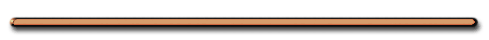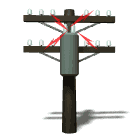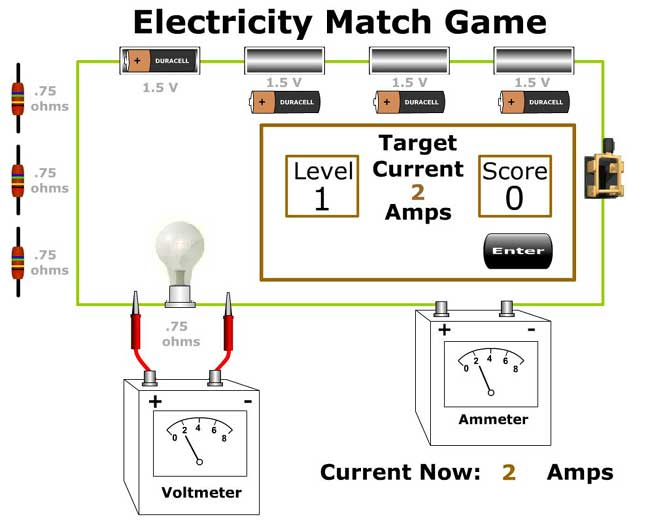HomeSeries, Parallel DifferencesIII) Electric Power

Power - time rate of doing work or expending energy

 P = W/t (watts) (J/seconds)

since V = W/q,

CROSS MULTIPLY

then W = Vq

P = Vq/t

 P = VI

(All equations in reference table)P = VI   since V = IR …

THEN P = I2R

since I = V/R …

THEN   P = V2/R

P = VI = I2R =  V2/R

P = W/t

Giancoli p. 516) 11

a) R = 20. ohms

P = W/t = VI

W = VIt

= 12V[.60 A]60 sec

b) 430 JEx) A series circuit contains two lamps and  a battery with a potential difference of 1.5 volts. If the current in the circuit is 0.10 ampere, at what rate does the circuit use energy?

Ex) Series circuit w/ two lamps, the battery supplies a potential difference of 1.5 volts. Current in the circuit is 0.10 ampere, what rate does the circuit use energy?a)15 W b)1.5 W d).15 W c).015W

V = 1.5 V
I = .10 Amps
P = ?

(rate of energy use)

P = VI = 1.5 V(.10 A)

P = .15 watts

or .15 VASeries Circuit Game - Flash

[ HTML5 ]
St. Mary H.S. PhysicsP, R Relationship????

P = I2R =  V2/R

 P,R direct when resistors have same current P = I2R P,R Inverse when resistors have same V P =  V2/R

 In which kind of circuit would a 5 Ω resistor be brighter (more power) than a 10 Ω resistor?   (Hint: P = V2/R and P = I2R)

Parallel Circuit, P = V2/R

P, R are inverse when
V is constant.

5 Ω would be brighter because it has less R

Review - Series or Parallel?Dorling Kindersley Books

Parallel

 AP Physics   p. 517) 33, 35, 37Electric EnergyEnrichmentAC Current - Tesla

Nikola Tesla - Mad Electricity part 3 of 5 - History Channel